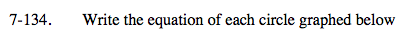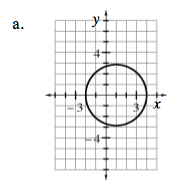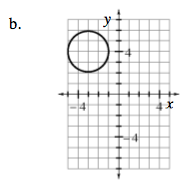### Home > AC > Chapter 13 > Lesson 13.OF1-S > Problem7-134

7-134.Recall the general equation of a circle: (xh)² + (yk)² = r² where (h, k) is the center of the circle and r is the radius.Write in graphing form:
(x − 1)2 + y2 = 9